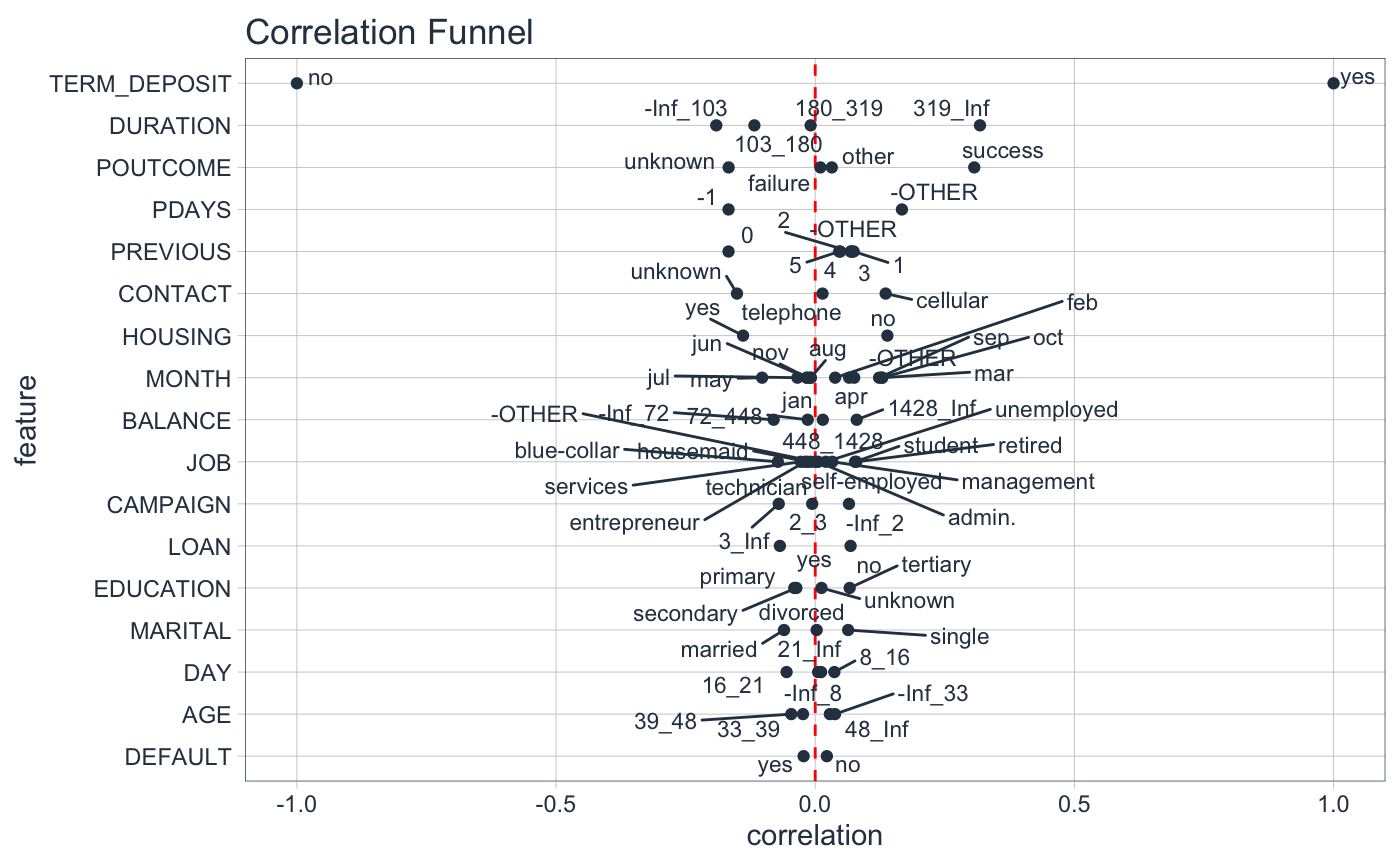plot_correlation_funnel returns a correlation funnel visualization in either static (ggplot2) or interactive (plotly) formats.

plot_correlation_funnel(
data,
interactive = FALSE,
limits = c(-1, 1),
alpha = 1
)

## Arguments

data A tibble or data.frame Returns either a static (ggplot2) visualization or an interactive (plotly) visualization Sets the X-Axis limits for the correlation space Sets the transparency of the points on the plot.

## Value

A static ggplot2 plot or an interactive plotly plot

binarize(), correlate()

## Examples

library(dplyr)
library(correlationfunnel)

marketing_campaign_tbl %>%
select(-ID) %>%
binarize() %>%
correlate(TERM_DEPOSIT__yes) %>%
plot_correlation_funnel()